## General Question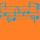# What are some good activites related to exponential equations that I can do with my 8th grade class?

Asked by dxs (15160) April 30th, 2016

They’re a pretty advanced class. They’ve learned about exponents and have a pretty good understanding. The goal now is to learn more about them. I was thinking of giving real-life examples, but I find it’s tricky to do at this level. Any fun activities you can think of that can get them thinking? Remember, they have already learned about exponential functions, we just need to go deeper into the topic. Feel free to be as creative as you want.

Observing members: 0Composing members: 0## 6 Answers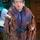I mean, exponential reproduction is pretty straightforward. Why not start with that?

trolltoll (2570)“Great Answer” (3) Flag as…This may be a bit advanced, but maybe you can show where e comes from, at least from an intuitive point of view. Suppose that a bank gives 100% interest. If you put \$1 in the bank, how much do you end up with at the end of the year, if you compound for n equally spaced intervals. You end up with (1 + 1/n) to the nth. In the limit this converges to e. You can have them check this on their calculators. You could then show that if the bank’s interest rate is i then the amount at the end of the year will be e to the i.

LostInParadise (28692)“Great Answer” (3) Flag as…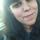I agree with @LostInParadise.

alcanzox (82)“Great Answer” (1) Flag as…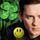Can you take them outside? With a ball and a atopwatch you can show them how long it takes an object to fall befoe hitting the ground. s=½ * a * t2. Displacement equals one half acceleration times the time squared.
You can have someone throw the ball up, another person can time it. Then divide that time by 2 ( up and down) and use the equation to calculate the height.

LuckyGuy (39449)“Great Answer” (3) Flag as…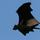Here is an introduction that many adults still have a hard time coming to terms with.

CWOTUS (26082)“Great Answer” (3) Flag as…Oooo!!! Show the effect of credit card interest and how the debt balloons!
Show how long it takes for a purchase made with a credit card at 29% APR will double the actual price of the item in fewer than 3 years. Casually mention the “rule of 72”. Divide 72 by the interest rate to get the number of years it takes to double the price. e.g. interest rate = 10% so the price will double in 72/10 = 7 years.
You’d be introducing them to exponents and giving them a couple of good, practical life lessons.
Extra credit:
What is the annual rate if the monthly rate is 2.0% per month? Much higher than 12×2!
2% seems so small but it adds up!

LuckyGuy (39449)“Great Answer” (1) Flag as…## Answer this questionor

#### Join

to answer.

This question is in the General Section. Responses must be helpful and on-topic.

Your answer will be saved while you login or join.
Have a question? Ask Fluther!
What do you know more about?
or
Knowledge Networking @ Fluther